# VBA Select Case

VBA Select Case statement is used instead of multiple Nested If statements. The VBA Select Case makes the VBA program easy to understand and faster in execution time.

The VBA Select Case statement is also called the Switch Case in many other languages such as Java, C++, C#, and JavaScript. It checks a variable for different values. If any case is true, then it will execute only that case and avoided the rest of other cases.

The Select Case statement is an alternative of the If Else If statement. It is another way to select a value from a list of values.

### Syntax

VBA Select Case statement follows the following syntax:

Explanation

• test_expression: It is a string or numeric value that comparing to the list of the conditions.
• condition_1 to condition_n: These are the conditions that evaluated in the order listed. If a condition is true, it will execute the corresponding code and not execute the further conditions of the code.
• Result_1 to result_n: The code that is executed when a condition is true.
• Case Else: If no condition is met to be accurate, then the else statement will be executed in the code.

### Examples

Here are some examples that help to understand the execution of the Select Case statement.

Example 1: We will find a condition where x = y with the help of the Select Case statement.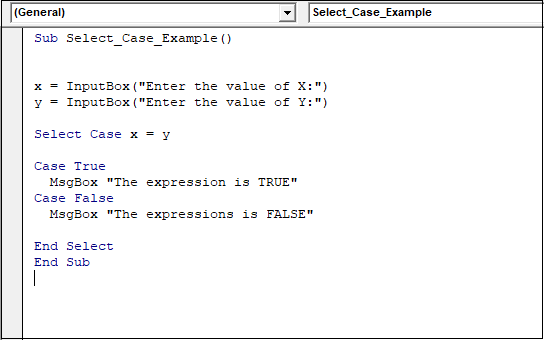If the condition is true, then the “Case True” block of the code will be executed. Otherwise, the “Case False” block of the code will be executed.

In the above example, we used the InputBox function to get values from the user.

Now execute the code and enter the values of X and Y.

Case 1: If the user enters both the different values of X and Y.Now press the OK button and enter the value for Y.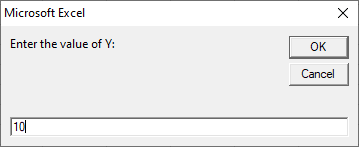You can see both the values of X and Y are different. After clicking on the OK button, it gives the following output.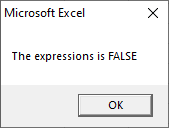Case 2: If the values of X and Y are equal.

Enter a value for X.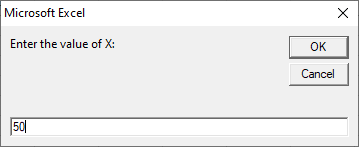Now click on the OK button, and enter the same value for Y.Again click on the OK button, and it gives the following output.Example 2: We want to check the entered number by the user is less than greater than or equal to 50.Here we used the IS keyword with a Case statement to compare values.

Now execute the code and give any value in between 1 to 100.Click on the OK button, and it gives the following output.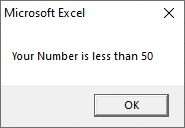Example 3: In this example, we check the multiple conditions within a single case with the help of the Select Case statement.This code will check the weather a number is even or odd when a user enters any number from 1 to 10.

We used (” “) to compare multiple conditions within a single Case statement.

Execute the code by using the Run button and enter any number start 1 to 10.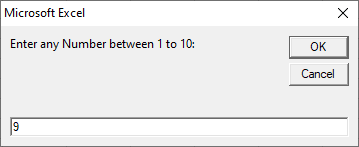Now click on the OK button it gives the following output.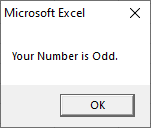Previous articleVBA Data Types
Next articleCircular reference in Excel